$\newcommand{\NN}{\mathbb{N}} \newcommand{\CC}{\mathbb{C}} \newcommand{\GG}{\mathbb{G}} \newcommand{\LL}{\mathbb{L}} \newcommand{\PP}{\mathbb{P}} \newcommand{\QQ}{\mathbb{Q}} \newcommand{\RR}{\mathbb{R}} \newcommand{\VV}{\mathbb{V}} \newcommand{\ZZ}{\mathbb{Z}} \newcommand{\FF}{\mathbb{F}} \newcommand{\KK}{\mathbb{K}} \newcommand{\UU}{\mathbb{U}} \newcommand{\EE}{\mathbb{E}} \newcommand{\Aa}{\mathcal{A}} \newcommand{\Bb}{\mathcal{B}} \newcommand{\Cc}{\mathcal{C}} \newcommand{\Dd}{\mathcal{D}} \newcommand{\Ee}{\mathcal{E}} \newcommand{\Ff}{\mathcal{F}} \newcommand{\Gg}{\mathcal{G}} \newcommand{\Hh}{\mathcal{H}} \newcommand{\Ii}{\mathcal{I}} \newcommand{\Jj}{\mathcal{J}} \newcommand{\Kk}{\mathcal{K}} \newcommand{\Ll}{\mathcal{L}} \newcommand{\Mm}{\mathcal{M}} \newcommand{\Nn}{\mathcal{N}} \newcommand{\Oo}{\mathcal{O}} \newcommand{\Pp}{\mathcal{P}} \newcommand{\Qq}{\mathcal{Q}} \newcommand{\Rr}{\mathcal{R}} \newcommand{\Ss}{\mathcal{S}} \newcommand{\Tt}{\mathcal{T}} \newcommand{\Uu}{\mathcal{U}} \newcommand{\Vv}{\mathcal{V}} \newcommand{\Ww}{\mathcal{W}} \newcommand{\Xx}{\mathcal{X}} \newcommand{\Yy}{\mathcal{Y}} \newcommand{\Zz}{\mathcal{Z}} \newcommand{\al}{\alpha} \newcommand{\la}{\lambda} \newcommand{\ga}{\gamma} \newcommand{\Ga}{\Gamma} \newcommand{\La}{\Lambda} \newcommand{\Si}{\Sigma} \newcommand{\si}{\sigma} \newcommand{\be}{\beta} \newcommand{\de}{\delta} \newcommand{\De}{\Delta} \renewcommand{\phi}{\varphi} \renewcommand{\th}{\theta} \newcommand{\om}{\omega} \newcommand{\Om}{\Omega} \renewcommand{\epsilon}{\varepsilon} \newcommand{\Calpha}{\mathrm{C}^\al} \newcommand{\Cbeta}{\mathrm{C}^\be} \newcommand{\Cal}{\text{C}^\al} \newcommand{\Cdeux}{\text{C}^{2}} \newcommand{\Cun}{\text{C}^{1}} \newcommand{\Calt}{\text{C}^{#1}} \newcommand{\lun}{\ell^1} \newcommand{\ldeux}{\ell^2} \newcommand{\linf}{\ell^\infty} \newcommand{\ldeuxj}{{\ldeux_j}} \newcommand{\Lun}{\text{\upshape L}^1} \newcommand{\Ldeux}{\text{\upshape L}^2} \newcommand{\Lp}{\text{\upshape L}^p} \newcommand{\Lq}{\text{\upshape L}^q} \newcommand{\Linf}{\text{\upshape L}^\infty} \newcommand{\lzero}{\ell^0} \newcommand{\lp}{\ell^p} \renewcommand{\d}{\ins{d}} \newcommand{\Grad}{\text{Grad}} \newcommand{\grad}{\text{grad}} \renewcommand{\div}{\text{div}} \newcommand{\diag}{\text{diag}} \newcommand{\pd}{ \frac{ \partial #1}{\partial #2} } \newcommand{\pdd}{ \frac{ \partial^2 #1}{\partial #2^2} } \newcommand{\dotp}{\langle #1,\,#2\rangle} \newcommand{\norm}{|\!| #1 |\!|} \newcommand{\normi}{\norm{#1}_{\infty}} \newcommand{\normu}{\norm{#1}_{1}} \newcommand{\normz}{\norm{#1}_{0}} \newcommand{\abs}{\vert #1 \vert} \newcommand{\argmin}{\text{argmin}} \newcommand{\argmax}{\text{argmax}} \newcommand{\uargmin}{\underset{#1}{\argmin}\;} \newcommand{\uargmax}{\underset{#1}{\argmax}\;} \newcommand{\umin}{\underset{#1}{\min}\;} \newcommand{\umax}{\underset{#1}{\max}\;} \newcommand{\pa}{\left( #1 \right)} \newcommand{\choice}{ \left\{ \begin{array}{l} #1 \end{array} \right. } \newcommand{\enscond}{ \left\{ #1 \;:\; #2 \right\} } \newcommand{\qandq}{ \quad \text{and} \quad } \newcommand{\qqandqq}{ \qquad \text{and} \qquad } \newcommand{\qifq}{ \quad \text{if} \quad } \newcommand{\qqifqq}{ \qquad \text{if} \qquad } \newcommand{\qwhereq}{ \quad \text{where} \quad } \newcommand{\qqwhereqq}{ \qquad \text{where} \qquad } \newcommand{\qwithq}{ \quad \text{with} \quad } \newcommand{\qqwithqq}{ \qquad \text{with} \qquad } \newcommand{\qforq}{ \quad \text{for} \quad } \newcommand{\qqforqq}{ \qquad \text{for} \qquad } \newcommand{\qqsinceqq}{ \qquad \text{since} \qquad } \newcommand{\qsinceq}{ \quad \text{since} \quad } \newcommand{\qarrq}{\quad\Longrightarrow\quad} \newcommand{\qqarrqq}{\quad\Longrightarrow\quad} \newcommand{\qiffq}{\quad\Longleftrightarrow\quad} \newcommand{\qqiffqq}{\qquad\Longleftrightarrow\qquad} \newcommand{\qsubjq}{ \quad \text{subject to} \quad } \newcommand{\qqsubjqq}{ \qquad \text{subject to} \qquad }$

Stein Unbiased Risk Estimator

# Stein Unbiased Risk Estimator

This tour uses the Stein Unbiased Risk Estimator (SURE) to optimize the value of parameters in denoising algorithms.

## Installing toolboxes and setting up the path.

You need to download the following files: signal toolbox and general toolbox.

You need to unzip these toolboxes in your working directory, so that you have toolbox_signal and toolbox_general in your directory.

For Scilab user: you must replace the Matlab comment '%' by its Scilab counterpart '//'.

Recommandation: You should create a text file named for instance numericaltour.sce (in Scilab) or numericaltour.m (in Matlab) to write all the Scilab/Matlab command you want to execute. Then, simply run exec('numericaltour.sce'); (in Scilab) or numericaltour; (in Matlab) to run the commands.

Execute this line only if you are using Matlab.

getd = @(p)path(p,path); % scilab users must *not* execute this


Then you can add the toolboxes to the path.

getd('toolbox_signal/');
getd('toolbox_general/');


## Denoising and SURE

We consider a simple generative model of noisy images $$F = f_0+W$$ where $$f_0 \in \RR^N$$ is a deterministic image of $$N$$ pixels, and $$W$$ is a Gaussian white noise distributed according to $$\Nn(0,\si^2 \text{Id}_N)$$, where $$\si^2$$ is the variance of noise.

The goal of denoising is to define an estimator $$h(F)$$ of $$f_0$$ that depends only on $$F$$, where $$h : \RR^N \rightarrow \RR^N$$ is a potentially non-linear mapping.

Note that while $$f_0$$ is a deterministic image, both $$F$$ and $$h(F)$$ are random variables (hence the capital letters).

The goal of denoising is to reduce as much as possible the denoising error given some prior knowledge on the (unknown) image $$f_0$$. A mathematical way to measure this error is to bound the quadratic risk $$\EE_W(\norm{h(F) - f_0}^2)$$, where the expectation is computed with respect to the distribution of the noise $$W$$

For real life applications, one does not have access to the underlying image $$f_0$$. In this tour, we however assume that $$f_0$$ is known, and $$f = f_0 + w\in \RR^N$$ is generated using a single realization of the noise $$w$$ that is drawn from $$W$$. We define the estimated deterministic image as $$h(f)$$ which is a realization of the random vector $$h(F)$$.

Number $$N = n \times n$$ of pixels.

n = 128*2;
N = n^2;


First we load an image $$f \in \RR^N$$ where $$N=n \times n$$ is the number of pixels.

name = 'hibiscus';
f0 = rescale( sum(load_image(name,n),3) );


Display it.

clf;
imageplot(f0);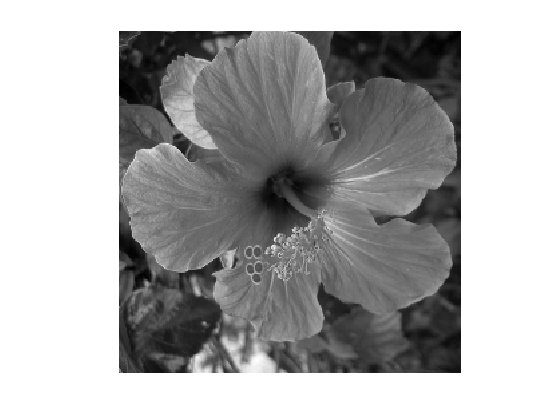Standard deviation $$\si$$ of the noise.

sigma = .08;


Then we add Gaussian noise $$w$$ to obtain $$f=f_0+w$$.

f = f0 + sigma*randn(n);


Display the noisy image. Note the use of the clamp function to saturate the result to $$[0,1]$$ to avoid a loss of contrast of the display.

clf;
imageplot(clamp(f), strcat(['Noisy, SNR=' num2str(snr(f0,f),3) 'dB']));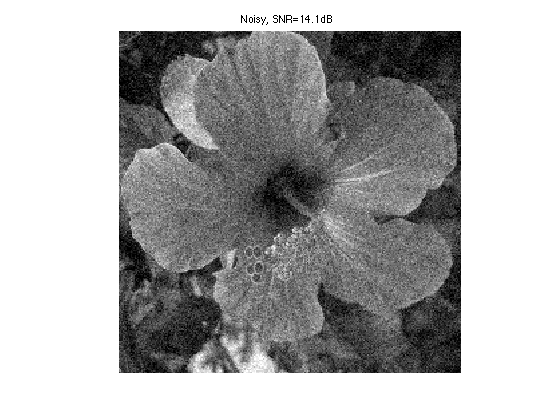The Stein Unbiased Risk Estimator (SURE) associated to the mapping $$h$$ is defined as $\text{SURE}(f) = -N\si^2 + \norm{h(f)-f}^2 + 2\si^2 \text{df}(f)$ where df stands for degree of freedom, and is defined as $\text{df}(f) = \text{div} h(f) = \sum_i \pd{h}{f_i}(f).$

It has been introduced in:

Stein, Charles M. (November 1981). "Estimation of the Mean of a Multivariate Normal Distribution". The Annals of Statistics 9 (6): 1135-1151.

And it has been applied to wavelet-based non-linear denoising in:

Donoho, David L.; Iain M. Johnstone (December 1995). "Adapting to Unknown Smoothness via Wavelet Shrinkage". Journal of the American Statistical Association (Journal of the American Statistical Association, Vol. 90, No. 432) 90 (432): 1200-1244.

If the mapping $$f \mapsto h(f)$$ is differentiable outside a set of zero measure (or more generally weakly differentiable), then SURE defines an unbiased estimate of the quadratic risk $\EE_W(\text{SURE}(F)) = \EE_W( \norm{f_0-h(F)}^2 ).$ This is especially useful, since the evaluation of SURE does not necessitate the knowledge of the clean signal $$f_0$$ (but note however that it requires the knowledge of the noise level $$\si$$).

In practice, one replaces $$\text{SURE}(F)$$ from its empirical evaluation $$\text{SURE}(f)$$ on a single realization $$f$$. One can then minimize $$\text{SURE}(f)$$ with respect to a parameter $$\la$$ that parameterizes the denoiser $$h=h_\la$$.

## Linear Denoising SURE

We consider a translation-invariant linear denoising operator, which is thus a convolution $h(f) = g \star h$ where $$g \in \RR^N$$ is a low pass kernel, and $$\star$$ denotes the periodic 2-D convolution.

Since we use periodic boundary condition, we compute the convolution as a multiplication over the Fourier domain. $\forall \om, \quad \hat h(f)(\om) = \hat f(\om) \hat g(\om)$ where $$\hat g(\om)$$ is the frequency $$\om$$ of the discrete 2-D Fourier transform of $$g$$ (computed using the function fft2).

convol = @(f,g)real(ifft2(fft2(f) .* repmat(fft2(g), [1 1 size(f,3)]) ));


We define a parameteric kernel $$g_\la$$ parameterized by its bandwidth $$\la>0$$. We use here a Gaussian kernel $g_\la(a) = \frac{1}{Z_\la} e^{ -\frac{\norm{a}}{2 \la^2} }$ where $$Z_\la$$ ensures that $$\sum_a g_\la(a) = 1$$.

normalize = @(f)f/sum(f(:));
x = [0:n/2 -n/2+1:-1];
[Y,X] = meshgrid(x,x);
g = @(lambda)normalize( exp( -(X.^2+Y.^2)/(2*lambda^2) ) );


Define our denoising operator $$h=h_\la$$ (we make explicit the dependency on $$\la$$): $h_\la(f) = g_\la \star f.$

h = @(f,lambda)convol(f, g(lambda));


Example of denoising result.

lambda = 1.5;
clf;
imageplot(clamp(h(f,lambda)));For linear operator, the dregree of freedom is equal to the trace of the operator, and thus in our case it is equal to the sum of the Fourier transform $\text{df}_\la(f) = \text{tr}(h_\la) = \sum_{\om} \hat g_\la(\om)$ Note that we have made explicit the dependency of df with respect $$\la$$. Note also that df$(f)$ actually does not depends on $$f$$.

df = @(lambda)real(sum(sum(fft2(g(lambda)))));


We can now define the SURE=SURE$$_\la$$ operator, as a function of $$f, h(f), \lambda$$.

SURE = @(f,hf,lambda)-N*sigma^2 + norm(hf-f, 'fro')^2 + 2 * sigma^2 * df(lambda);


Exercice 1: (check the solution) For a given $$\lambda$$, display the histogram of the repartition of the quadratic error $$\norm{y-h(y)}^2$$ and of $$\text{SURE}(y)$$. Compute these repartition using Monte-Carlo simulation (you need to generate lots of different realization of the noise $$W$$. Display in particular the location of the mean of these quantities.

exo1;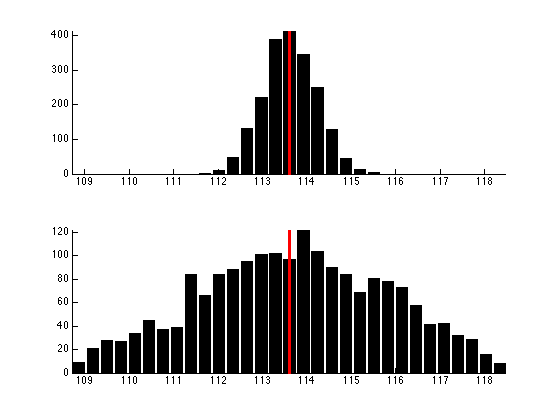In practice, the SURE is used to set up the value of $$\la$$ from a single realization $$f=f_0+w$$, by minimizing $$\text{SURE}_\la(f)$$.

Exercice 2: (check the solution) Compute, for a single realization $$f=f_0+w$$, the evolution of $E(\la) = \text{SURE}_\la(f) \qandq E_0(\lambda) = \norm{f-h_\la(f)}^2$ as a function of $$\lambda$$.

exo2;Exercice 3: (check the solution) Display the best denoising result $$h_{\la^*}(f)$$ where $\la^* = \uargmin{\la} \text{SURE}_\la(f)$

exo3;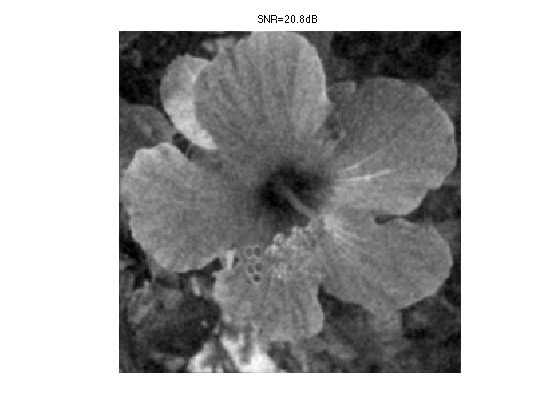## Soft Thresholding SURE

In order to enhance the denoising results for piecewise regular signal and image, it is possible to use non-linear thresholding in an orthogonal wavelet basis $$\Bb = \{ \psi_m \}_{m}$$ where $$\psi_m \in \RR^N$$ is a wavelet element.

Re-generate a noisy image.

f = f0 + sigma*randn(n);


The soft-thresholding estimator thus reads $h_\la(f) = \sum_m s_\la( \dotp{f}{\psi_m} ) \psi_m \qwhereq s_\la(\al) = \max\pa{0, 1-\frac{\la}{\abs{\al}}} \al.$ It can be conveniently written as $h_\la = \Ww^* \circ S_\la \circ \Ww$ where $$\Ww$$ and $$\Ww^*$$ are forward and inverse wavelet transform $\Ww(f) = ( \dotp{f}{\psi_m} )_m \qandq \Ww^*(x) = \sum_m x_m \psi_m,$ and $$S_\la$$ is the diagonal soft thresholding operator $S_\la(x) = ( s_\la(x_m) )_m.$

Define the wavelet transform and its inverse.

W  = @(f)perform_wavortho_transf(f,0,+1);
Ws = @(x)perform_wavortho_transf(x,0,-1);


Display the wavelet transform $$\Ww(f_0)$$ of the original image.

clf;
plot_wavelet(W(f0),1);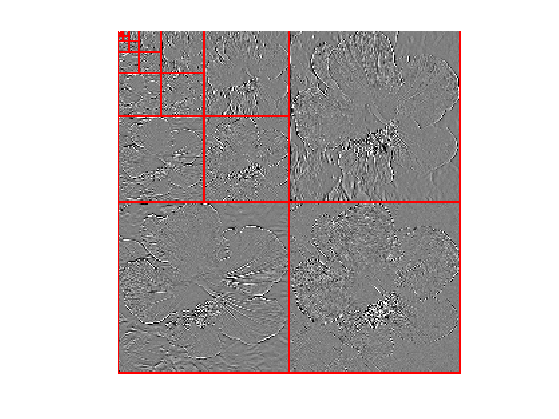Define the soft thresholding operator.

S = @(x,lambda)max(0, 1-lambda ./ max(1e-9,abs(x)) ) .* x;


Define the denoising operator.

h = @(f,lambda)Ws(S(W(f),lambda));


Example of denoising result.

lambda = 3*sigma/2;
clf;
imageplot(clamp(h(f,lambda)));Since $$Ww$$ is an orthogonal transform, one has $\text{df}(f) = \text{div}( S_\la )( \Ww(f) ) = \sum_m s_\la'( \dotp{f}{\psi_m} ) = \norm{\Ww(h(f))}_0$ where $$s_\la'$$ is the derivative of the 1-D function $$s_\la$$, and $$\norm{\cdot}_0$$ is the $$\ell^0$$ pseudo-norm $\norm{x}_0 = \abs{ \enscond{m}{x_m \neq 0} }.$

To summerize, the degree of freedom is equal to the number of non-zero coefficients in the wavelet coefficients of $$h(f)$$.

df = @(hf,lambda)sum(sum( abs(W(hf))>1e-8 ));


We can now define the SURE operator, as a function of $$f, h(f), \lambda$$.

SURE = @(f,hf,lambda)-N*sigma^2 + norm(hf-f, 'fro')^2 + 2 * sigma^2 * df(hf,lambda);


Exercice 4: (check the solution) For a given $$\lambda$$, display the histogram of the repartition of the quadratic error $$\norm{y-h(y)}^2$$ and of $$\text{SURE}(y)$$. Compute these repartition using Monte-Carlo simulation (you need to generate lots of different realization of the noise $$W$$. Display in particular the location of the mean of these quantities. Hint: you can do the computation directly over the wavelet domain, i.e. consider that the noise is added to the wavelet transform.

exo4;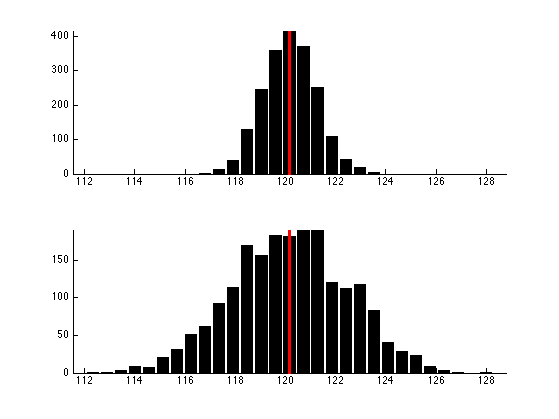Exercice 5: (check the solution) Compute, for a single realization $$f=f_0+w$$, the evolution of $E(\la) = \text{SURE}_\la(f) \qandq E_0(\lambda) = \norm{f-h_\la(f)}^2$ as a function of $$\lambda$$.

exo5;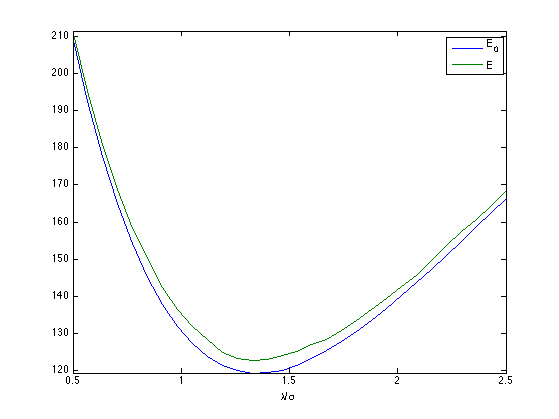Exercice 6: (check the solution) Display the best denoising result $$h_{\la^*}(f)$$ where $\la^* = \uargmin{\la} \text{SURE}_\la(f)$

exo6;## Block-soft Thresholding SURE

To improve the result of soft thresholding, it is possible to threshold blocks of coefficients.

We define a partition $$\{1,\ldots,N\} = \cup_k b_k$$ of the set of wavelet coefficient indexes. The block thresholding is defined as $h_\la(f) = \sum_k \sum_{m \in b_k} a_\la( e_k ) \dotp{f}{\psi_m} \psi_m \qwhereq e_k = \sum_{m \in b_k} \abs{\dotp{f}{\psi_m}}^2,$ where we use the James-Stein attenuation threshold $a_\la(e) = \max\pa{ 0, 1 - \frac{\la^2}{e^2} }.$

The block size $$q$$.

q = 4;


A function to extract blocks.

[dX,dY,X,Y] = ndgrid(0:q-1,0:q-1,1:q:n-q+1,1:q:n-q+1);
I = X+dX + (Y+dY-1)*n;
blocks = @(fw)reshape(fw(I(:)),size(I));


A function to reconstruct an image from blocks.

linearize = @(x)x(:);
unblock = @(H)reshape( accumarray( I(:), linearize(H), [n*n 1], @min), [n n]);


Compute the average energy of each block, and duplicate.

energy = @(H)mean(mean(abs(H).^2,1),2);
energy = @(H)repmat( max3(energy(H),1e-15), [q q]);


Threshold the blocks. We use here a Stein block thresholding. All values within a block are atenuated by the same factor.

S = @(H,lambda)max(1-lambda^2 ./ energy(H),0) .* H;


Block thresholding estimator $$h_\lambda(f)$$.

h = @(f,lambda)Ws(unblock(S(blocks(W(f)),lambda) ) );


Example of block denoising.

lambda = 1.1*sigma;
clf;
imageplot(clamp(h(f,lambda)));Since the block-thresholding operates in a block diagonal manner over the wavelet coefficients, it degree of freedom is a sum of the divergence of each block James-Stein operator $\text{df}(f) = \sum_{ e_k > \la^2 } \text{tr}( \partial \phi (a_k) )$ where $$a_k = (\dotp{f}{\psi_m})_{m \in b_k}$$ is the set of coefficients inside a block, that satisfies $$\norm{a_k}=e_k$$, and where $\phi(a) = \pa{ 1 - \frac{\la^2}{\norm{a}^2} } a.$ One can compute explicitely the derivative of $$\phi$$ $\partial \phi(a) = \pa{ 1 - \frac{\la^2}{\norm{a}^2} } \text{Id} + 2 \frac{\la^2}{\norm{a}^2} \Pi_a$ where $$\Pi_a$$ is the orthogonal projector on $$a$$.

This gives the folowing formula for the degree of freedom $\text{df}(f) = \norm{\Ww(h_\la(f))}_0 + \sum_{ e_k > \la^2 } \frac{\la^2}{e_k} (2-\abs{b_k}).$ One can note that the degree of freedom differs from the one of the soft thresholding (it is not in general an integer).